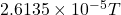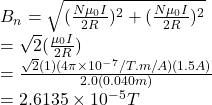## Two circular loops of wire, each containing a single turn, have the same radius of 5.1 cm and a common center. The planes of the loops are p

Question

Two circular loops of wire, each containing a single turn, have the same radius of 5.1 cm and a common center. The planes of the loops are perpendicular. Each carries a current of 1.5 A. What is the magnitude of the net magnetic field at the common center

in progress 0
2 weeks 2021-08-29T18:45:40+00:00 1 Answers 0 views 0Hence, the net magnitude at the common center is